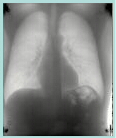Aprend TechnologyEnergy-selective x-ray imaging and other topics

Topics covered are:
X-ray imaging
Energy Selective x-ray imaging
Computer-assisted diagnosis (future)
Image Analysis and computer vision (future)

A free ebook on the topics discussed in my blog is available. The ebook puts together and organizes all the blog posts in one document. This makes it easier to search for all discussions of a particular topic and to see the relatioships between the posts. There is a table of contents and the pdf has "bookmarks" so it is easy to jump from post to post. So far, the book is over 260 pages.

To get it, email me atThe book is revised periodically. If you want an updated version, email me at the address above.

Latest Revision:

May 2, 2015Monte Carlo simulation of SNR with energy information

SNR with energy information

CT projection Simulator--2

A projection simulator--Matlab implementation

Curve intersection

Interection of Line Segments

A Line object--basic formulas

The uses of complex variables in Matlab

Matlab interface to the projection simulator

A projection simulator for image reconstruction research--implementation

A projection simulator for image reconstruction research--rationale

An improved parallel projection image reconstructor for Matlab

The dimensionality of attenuation coefficients

Matrices, the singular value decomposition, and energy-selective imaging

A Matlab function to compute the attenuation coefficient

Cross-section and Attenuation Coefficient Physics